# Crack IBPS PO : Aptitude day 79 : new pattern Quadratic Equation

D.1 – 5) In the following question two quantities are given Quantity 1 and quantity 2 By solving those quantities give corresponding answer.

1) Quantity 1 : Find the minimum possible profit % if CP of 1 apple is equal to CP of 2 orange and SP of 1 apple is equal to CP of 3 orange. No fruit is sold below the CP. There are 8 apples & 5 oranges.

Quantity 2 : By selling 27 articles a man gains cost price of 9 articles. Find the profit % ?

a) Quantity 1 ≥ Quantity 2

b) Quantity 1 = Quantity 2 (or) No relation

c) Quantity 1 > Quantity 2

d) Quantity 1 ≤ Quantity 2

e) Quantity 1 < Quantity 2

e) Quantity 1 < Quantity 2

Explanation:

Quantity I :

Let CP of 1 orange be Re. 1

CP of 1 apple = CP of 2 orange = Rs. 2

CP of 8 apple = Rs. 16

SP of 1 apple = CP of 3 orange = Rs. 3

SP of 8 apple = Rs. 24

Say, CP of 5 orange = SP of 5 orange = Rs. 5

Total CP = 16 + 5 = Rs. 21

Total SP = 24 + 5 = Rs. 29

Minimum possible profit % = (SP-CP)/CP × 100 = (29-21)/21 × 100 = 38.09%

Quantity II :

Let CP of 1 article be Re. 1

Then CP of 27 article = Rs. 27

Profit By earning 27 articles = CP of 9 articles = Rs. 9

SP of 27 articles = CP of 27 articles + CP of 9 articles = Rs. 36

Profit % = (SP-CP)/CP×100 = (36-27)/27×100 = 33 1/3 %

Hence Quantity 1>Quantity 2

2)Quantity 1 : Train 1 starts from station A to reach station B and Train 2 starts from station B to reach station A. Train 1 & Train 2 takes 7hrs & 8rs respectively to reach their destination. After how much time will both trains meet each other if they started at same time ?

Quantity 2 : Two train are running at speed of 60 km/hr& 70km/hr in opposite directions. At what time both trains meet each other if the distance between them is 4,55,000mts.

a) Quantity 1 ≥ Quantity 2

b) Quantity 1 = Quantity 2 (or) No relation

c) Quantity 1 > Quantity 2

d) Quantity 1 ≤ Quantity 2

e) Quantity 1 < Quantity 2

c) Quantity 1 > Quantity 2

Explanation:

Quantity I:

Time taken by Train 1 & Train 2 to reach their destination is 7hrs & 8hrs respectively.

Assume distance between two train is 56 Km LCM of 7hrs & 8hrs

Time at which both trains meet each other is,

= 56/15 × 60 = 224 Mins

Quantity II :

Distance between two trains = 455000/1000 = 455 Kms

Time at which both trains meet each other are running in opposite directions,

= 455/(60+70) = 455/130×60= 210 mins

Hence Quantity 1> Quantity 2

3) Quantity 1:

Four men take 30 days to complete two third of the work. How many days will a single man take to complete the work?

Quantity 2:Ten men and twelve women can complete a piece of work in 30 days while 4 men and 4 women can complete the same piece of work in 72 days. In how many days 2 men can complete the work?

a) Quantity 1 ≥ Quantity 2

b) Quantity 1 = Quantity 2 (or) No relation

c) Quantity 1 > Quantity 2

d) Quantity 1 ≤ Quantity 2

e) Quantity 1 < Quantity 2

c) Quantity 1 > Quantity 2

Quantity 1:

No of men working in case 1=4

No of days they take to two third of work=30

To complete the work, they may take45 days.

The work of 4 men in a day = 1/45

The work of a single man in a day = 1/(45×4)=1/180

The man will complete the work in 180 days.

Quantity 2:

10x+12y = 1/30 → (I)

4x+4y= 1/72 → (II)

Solving the both equations,

A man can complete a in 240 days.

So, two men complete a work in 120 days.

Comparing the two results, 180>120 i.e, x>y

4)Quantity 1:Raghu invested a certain sum in Scheme X for 4 years. Scheme X offers simple interest at 12% p.a. for the first two years and compound interest (compounded annually) at 20% p.a. for the next two years. The total interest earned by him after 4 years is Rs. 11016. What was the sum invested by Raghu in Scheme X?

Quantity 2:The compound interest accrued on an amount at the end of 2 year at the rate of 12% p.a. is Rs. 2862. What is the amount?

a) Quantity 1 ≥ Quantity 2

b) Quantity 1 = Quantity 2 (or) No relation

c) Quantity 1 > Quantity 2

d) Quantity 1 ≤ Quantity 2

e) Quantity 1 < Quantity 2

c) Quantity 1 > Quantity 2

Quantity 1: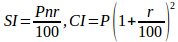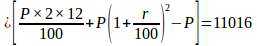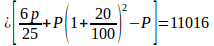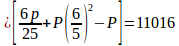By solving we get =16,200

Quantity 2:

For 2 years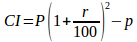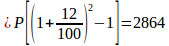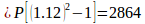By solving we get

P=11250

Hence Quantity 1>Quantity2

5)Quantity 1:A man, a women and a boy can complete a piece of work in 6 days 9 days and 18 days respectively. How many must assist 1 men and 2 women so as to complete the work in 2 days

Quantity 2: A man, a women and a boy can complete a piece of work in 3 days 4 days and 12 days respectively. How many must assist 1 men and 1 women so as to complete the work in 1/4 days

a) Quantity 1 ≥ Quantity 2

b) Quantity 1 = Quantity 2 (or) No relation

c) Quantity 1 > Quantity 2

d) Quantity 1 ≤ Quantity 2

e) Quantity 1 < Quantity 2

e) Quantity 1 < Quantity 2

Explanation:

Quantity 1:

1men and 2women one day work=(1×3)+(2×2)=7 units

In 2 days 14 units

By taking LCM we get Total work =18

remaining work=18-14=4

the work will be completed by boys in 2 days

Therefore 2 boys will be required to complete the work

Quantity 2:

By taking LCM we get total work=12 units

Efficiency of man=4

women=3

Boy=1

They have to complete the work in 1/4 day means 4×12=48 units

Men + Women efficiency=4+3=7 units

Remaining units=48-7=41 done by boys

41/1=41boys

Hence quantity 1<Quantity 2

D.6-10) In the following question two quantities are given Quantity 1 and quantity 2 By solving those quantities give corresponding answer.

6) Quantity 1:The driver of the Maruti car driving at the speed of 68 km/hr locates a bus 40 metres ahead of him travelling in the same direction. After 10 seconds the bus is 60m behind. The speed of the bus is

Quantity 2:Two guns were fired from the same place at an interval of 15min,but a person in a bus approaching the place hears the second report 14min 30 sec after the first. Find the speed of the bus supposing that sound travels 330m per sec

a) Quantity 1 ≥ Quantity 2

b) Quantity 1 = Quantity 2 (or) No relation

c) Quantity 1 > Quantity 2

d) Quantity 1 ≤ Quantity 2

e) Quantity 1 < Quantity 2

e) Quantity 1 < Quantity 2

Quantity 1:

Let speed be S

In 10 sec car covers the relative distance

=60+40=100m

Relative Speed=100/10=10m/s

=10×18/5=36 km/hr

=68-S=36

S=32 km/hr

Quantity 2:

Distance travelled by the bus in 14min30sec could be travelled by sound in(15-14min 30 sec)=30second

Bus travels=330×30min in 14 1/2 min

Speed of the bus per hour

=(330×30×2×60)/(29×1000)=(99×12)/29=1188/29=4028/(29 ) km/hr

7) Quantity 1:The radii of circular ends of frustum of height 10cm and 22cm and 5cm respectively. Find the Lateral surface area

Quantity 2:Find the surface area of a sphere of radius 11cm

a) Quantity 1 ≥ Quantity 2

b) Quantity 1 = Quantity 2 (or) No relation

c) Quantity 1 > Quantity 2

d) Quantity 1 ≤ Quantity 2

e) Quantity 1 < Quantity 2

e) Quantity 1 < Quantity 2

Explanation:

Quantity 1:

R=22cm,r=10cm,h=5cm

L=Slant height

l=√((R-r)2+h2 )

l=√((22-10)2+52 )

l=√(144+25)

l=13cm

Lateral surface area=π(R+r)l

=π(22+10)13

=π(416)

=1307.42cm2

Quantity 2:

surface area=4πr2

=4π×121

=1521.14cm2

Hence Quantity 1 < Quantity 2

8) Quantity 1: The Volume of a cube whose surface area is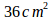Quantity 2: Find the area of the quadrilateral piece of ground one of whose diagonal is 11 cm long and the perpendicular from the other two vertices are 9cm and 7cm respectively.

a) Quantity 1 ≥ Quantity 2

b) Quantity 1 = Quantity 2 (or) No relation

c) Quantity 1 > Quantity 2

d) Quantity 1 ≤ Quantity 2

e) Quantity 1 < Quantity 2

e) Quantity 1 < Quantity 2

Quantity 1:

Volume of the cube=(√((Surface area)/6))3=(√(36/6))3

=23=8cm2

Quantity 2:

Area of the quadrilateral = 1/2×d×(h1+h2)

d=11cm

h1=9cm

h2=7cm

Area=1/2×11(7+9)

=1/2×11(16)

=176/2=88cm2

Hence Quantity 1< Quantity 2

9)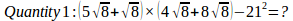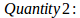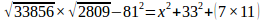a) Quantity 1 ≥ Quantity 2

b) Quantity 1 = Quantity 2 (or) No relation

c) Quantity 1 > Quantity 2

d) Quantity 1 ≤ Quantity 2

e) Quantity 1 < Quantity 2

c) Quantity 1 > Quantity 2

Explanation:

Quantity 1:

(5√8+√8)×(4√8+8√8)-212=?

(20×8)+(40×8)+(4×8)+(8×8)-441=?

=160+320+32+64-441

=135

Quantity 2:(184×53)-6561=?2+1089+77

x2=9752-6561-1089-77

x2=2025

x=√2025=45

Hence Quantity 1>Quantity 2

10)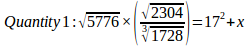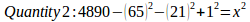a) Quantity 1 ≥ Quantity 2

b) Quantity 1 = Quantity 2 (or) No relation

c) Quantity 1 > Quantity 2

d) Quantity 1 ≤ Quantity 2

e) Quantity 1 < Quantity 2

a) Quantity 1 ≥ Quantity 2

Quantity 1: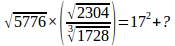76× 48/12 = 289 +?

304=289+?

X = 15

Quantity 2:

652=4225

212=441

4890-4225=665

665-441=224

224+1=225

x2=225

x=±15

Hence Quantity 1≥Quantity 2